# Math in Focus Grade 2 Chapter 1 Practice 3 Answer Key Comparing Numbers

Go through the Math in Focus Grade 2 Workbook Answer Key Chapter 1 Practice 3 Comparing Numbers to finish your assignments.

## Math in Focus Grade 2 Chapter 1 Practice 3 Answer Key Comparing Numbers

Write greater than or less than in the blanks.

Example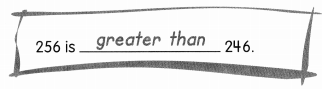First compare the hundreds. If the hundreds are the same, compare the tens. If the tens are the same, compare the ones.Question 1.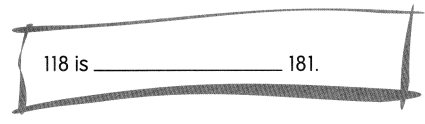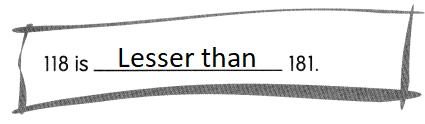Explanation:
First compared the hundreds.
As the hundreds are the same, compared the tens.
181 > 118

Question 2.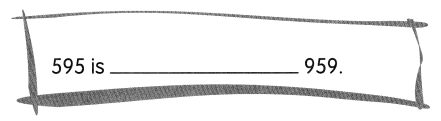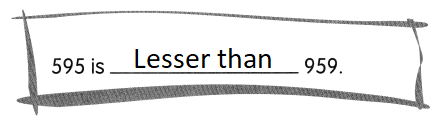Explanation:
Compared the hundreds as the hundreds are different
959 > 595

Compare.
Write > or < in the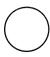.

Example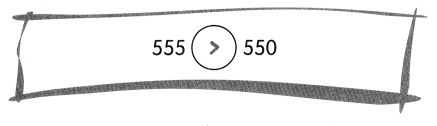Write > or < in the.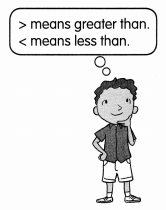Question 3.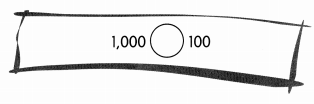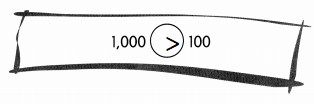Explanation:
thousands are greater than hundreds

Question 4.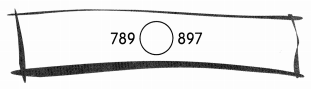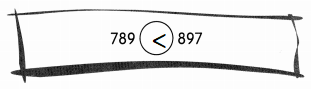Explanation:
compared the hundreds as the hundreds are different
897 > 789

Question 5.
Write T for true or F for false.
55 is less than 455. ____
355 is greater than 455. _______
400 is less than 455. ______
450 is greater than 405. ______
800 < 809 ______
980 < 809 ____
89 > 98 ____
863 > 862 ____
55 is less than 455.  T
55 < 455
355 is greater than 455. F
355 < 455
400 is less than 455. T
400 < 455
450 is greater than 405. T
450 > 405
800 < 809 T
800 is less than 809
980 < 809 F
980 is greater than 809
89 > 98 F
89 is lesser than 98
863 > 862 T
863 is greater than 862